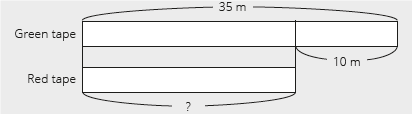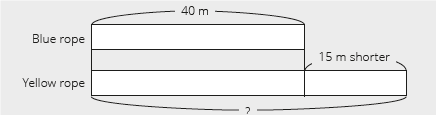# Lesson Notes By Weeks and Term - Primary 2

Word problems

TERM: 2nd Term

WEEK: 10

CLASS: Primary 2

AGE: 7 years

DURATION: 5 periods of 40 minutes each

DATE:

SUBJECT: Mathematics

TOPIC: Word problems

SPECIFIC OBJECTIVES: At the end of the lesson, the pupils should be able to

1. Solve addition word problems using brackets to simplify number sentences.
2. Solve word problems by looking at the difference.
3. Solve problems looking at the first amount.

INSTRUCTIONAL TECHNIQUES: Explanation, question and answer, demonstration, practical

INSTRUCTIONAL MATERIALS: Bottle tops

 PRESENTATION TEACHER’S ACTIVITY PUPIL’S ACTIVITY STEP 1MENTAL MATHS The teacher begins the lesson with some mental calculationsCalculate1 23 + 10 =  2 35 + 20 =  3 36 + 30 =  4 38 + 40 =  5 47 + 50 =  6 30 + 23 =7 20 + 45 =8 60 + 36 =9 40 + 32 =10 20 + 68 = Pupils respond and participate STEP 2CONCEPTDEVELOPMENT The teacher• Writes the following word problem on the board. There are 18 cars in the parking.4 cars came in and6 cars came in later.How many cars are there in the parking? The word problem must be written in several rows divided to assist learners to identify the critical information/numbers needed to solve the problem.• Reads the problem.• Lets learners read the problem until they read it fluently.• Underlines the numbers, 18, 4 and 6.• Underlines the question (How many cards does she have now?) with a wavy line.• Lets learners manipulate bottle tops to represent the story.• Then, lets them draw circles in their classwork book (with words explaining it) as follows.• Draws a diagram as above.This is a special case diagram. This diagram helps learners focus on the numbers to add to the first number. Hence, teachers can draw it and learners copy it.• Lets the learners draw the diagram.• Lets learners determine the operation from the bar diagram and write the number sentence.• Lets learners present their number sentence and confirm with the class the correct number sentence.• Lets the learners solve the number sentence (18 + 4 + 6 = 28).• Asks: How did you do the calculation? (a. 18 + 4 = 22, then 22 +6 =28 or b. 4 + 6 = 10,18 + 10 = 28).• Introduces bracket that shows adding the numbers in bracket first, i.e. 18 + (4 + 6) =18 + 10 =28.• Asks: What is the answer for the word problem? (There are 28 cars now).Learners have to answer with unit, 28 cars. CLASS ACTIVITYThe teacher• Writes the following word problem on the board: There were 17 ducks in the pond.6 more ducks came andanother 4 came later.How many ducks are there altogether? • Using the steps from activity 1 solve this word problem with the learners.17 + 6 + 4 = 17 + (6 + 4) = 17 + 10 = 27, 27 ducks Pupils pay attention and participate STEP 3CLASS-WORK 1 Solve the following problems. Remember to start with the brackets.a 14 + (7 + 3) = ___  b 25+ ( 3 + 2) = ___c 19 + (2 + 18) = ___d 24 + (17 + 3) = ___e 35+ (14 + 6) = ___ f 49 + (8+22) = ___ 2 Use brackets to solve this problem.There were 16 dogs in the park.7 more dogs came andanother 3 came later.How many dogs are there altogether? Pupils attempt their class work STEP 4HOME-WORK Solve the following problems. Remember to solve the brackets first.a 15+ (5+5) = ___b 19 + (4 + 6) = ___c 27 + (12+8) = ___d 37 + (46 + 4) = ___ The pupils writes it in their homework book STEP 5SUMMARY The teacher summarizes by reminding the pupils that in this lesson we have learnt to solve addition word problems using brackets. She marks their class works, makes corrections where necessary and commends them positively

PERIOD 2: Assessment

 PRESENTATION TEACHER’S ACTIVITY PUPIL’S ACTIVITY STEP 1ORALASSESSMENTS The teacher asks questions on mental maths treated so far Pupils respond and participate STEP 2DISCUSSION The teacher discusses all the methods used by some learners in the oral assessments(some of the questions are solved on the board by the learners) and addresses any misconceptions that may have risen Pupils pay attention and participate STEP 3WRITTEN ASSESSMENTS 1 Solve the following. Remember start with the brackets.a 20 + (4 + 6) = ___b 40 + (5 + 5) = ___c 43 + (30 + 10) = ___ 2 Use two different methods to solve each problem.a There were 15 books on the shelf.I put in 3 more books on the shelf and thenI put another 7 books.How many books do I have altogether? b There were 25 oranges in a basket.Mom bought 7 more oranges and thenshe bought another 3 oranges.How many oranges are there now? c There were 15 horses in the field.7 more horses came to the field and then5 horses left the field.How many horses are left in the field? Pupils attempt their class work STEP 4SUMMARY The teacher marks the written assessments, corrects were necessary and commends the pupils

PERIOD 3: Word problems-difference

 PRESENTATION TEACHER’S ACTIVITY PUPIL’S ACTIVITY STEP 1MENTAL MATHS The teacher begins the lesson with some mental calculationsCalculate1 51 – 10 =2 49 – 20 =  3 62 – 50 =4 75 – 30 =5 99 – 20 =  6 55 – 40 =7 88 – 70 =8 66 – 30 =9 73 – 50 =  10 81 – 20 = Pupils respond and participate STEP 2CONCEPTDEVELOPMENT The teacher• Writes the following word problem on the board: There are red and green tapes.The green tape is 35 m.The green tape is 10 m longer than the red tape.How long is the red tape?(35 – 10 = 25, 25 m) Pupils pay attention and participate STEP 3CLASS-WORK Note: Do not erase the workings for Activity 1. The learners will need these to guide them with their classwork. Draw bar diagrams to solve the problems below. a There are 22 sheep in the field.There are 6 more sheep in the field than on the truck.How many sheep are there on the truck? b A dozen pencils costs N45.This is N15 more expensive than a packet of erasers.How much is a packet of erasers? Pupils attempt their class work STEP 4HOME-WORK Draw a bar diagram to solve the problems below.There are 38 girls on the playground.There are 20 more girls than boys on the playground.How many boys are there? The pupils writes it in their homework book STEP 5SUMMARY The teacher summarizes by reminding the pupils that in today’s lesson we have learnt to solve word problems by looking at the difference. She marks their class works, makes corrections where necessary and commends them positively

PERIOD 4: Word problems-comparison

 PRESENTATION TEACHER’S ACTIVITY PUPIL’S ACTIVITY STEP 1MENTAL MATHS The teacher begins the lesson with some mental calculationsCalculate1 15 + 10 =2 16 + 20 =  3 34 + 30 =  4 38 + 40 =  5 22 + 60 =  6 30 + 21 =7 20 + 42 =8 20 + 33 =9 30 + 47 =10 40 + 29 = Pupils respond and participate STEP 2CONCEPTDEVELOPMENT The teacher• Writes the following word problem on the board. There is a blue and a yellow rope.The blue rope is 40 m.The blue rope is15 m shorter than the yellow rope.How long is the yellow rope? The word problem must be written on four lines as shown above to assist learners to identify the critical information/numbers needed to solve the problem. • Reads the problem.• Lets learners read the problem until they read it fluently.• Underlines the numbers, 40 and 15.• Underlines the question (How long is the yellow tape?) with a wavy line.• Lets learners draw bar diagram to represent the story.• Lets some learners present their diagrams.• Lets learners determine the operation from the bar diagram and write the number sentence.Avoid teaching to determine the operation by keywords, e.g. if there is a word ‘less’ in the word problem, it is subtraction. This is not always true.• Lets learners present their number sentence and confirm with the class the correct number sentence.• Lets the learners solve the number sentence (40 + 15 = 55).• Asks: What is the answer for the word problem? (The yellow rope is 55 m.)Learners have to answer with unit, 45 m. Pupils pay attention and participate STEP 3CLASS-WORK Note: Do not erase the workings for Activity 1. The learners will need these to guide them with their classwork. Draw bar diagrams to solve the problems below. a There are 29 taxis on the road.The number of taxis is 10 less than the cars.How many cars are there? b A red ribbon is 20 m long.It is 36 m shorter than a blue ribbon.How long is the blue ribbon? Pupils attempt their class work STEP 4HOME-WORK Draw a bar diagram to solve the problem.There are 17 oranges.There are 30 less oranges than apples.How many apples are there? The pupils writes it in their homework book STEP 5SUMMARY The teacher summarizes by reminding the pupils that in today’s lesson we have learnt to solve problems looking at the first amount. She marks their class works, makes corrections where necessary and commends them positively

PERIOD 5: Weekly Test/consolidations

TEACHER’S ACTIVITY: The teacher revises all the concepts treated from period 1-4 and gives the pupils follow through exercises, quiz and tests. She marks the exercises, makes corrections and commends the pupils positively.

PUPIL’S ACTIVITY: The pupils work on the worksheets and exercises give by the teacher individually

CONSOLIDATION

Draw bar diagrams to show the difference.

1 There are 18 pigs on the farm.

There are 5 more pigs than goats.

How many goats are there?

2 There are 25 books on the shelf.

There are 10 more books than magazines.

How many magazines are there?

3 There are 30 plates on the table.

There are 16 less plates than spoons on the table.

How many spoons are there?

4  A cup of rice costs N20.

A cup of rice is N15 cheaper than a cup of beans.

How much is a cup of beans?